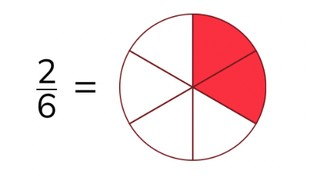Writing a fraction in a circle diagram

# Writing a fraction in a circle diagram

Students learn to write a fraction in a circle diagram.8,000 schools use Gynzy92,000 teachers use Gynzy1,600,000 students use Gynzy

## General

The students learn how they can write a fraction in a circle diagram.

## Standards

CCSS.Math.Content.3.NF.A.1

## Learning objective

Students will be able to write simple fractions in a circle diagram.

## Introduction

Have the students listen to each of the speakers. They must write down the fraction that they hear. Have them hold up their answers so that you can check whether they have written down the correct fraction. Next the students work in pairs to say each fraction that they see out loud.

## Instruction

Explain that a fraction is made up of a numerator and a denominator. After this you explain through an example how you can write a fraction in a circle diagram. First see how many parts you need to divide the circle diagram up into. The denominator tells this. Next you divide the circle diagram into this number of equal parts. Then you see how many parts need to be colored in. The numerator tells this. Then you can color in that number of parts. After this, you show an image with an accompanying fraction. The students must fill in a circle diagram to the given fraction. Click on the sections in the circle diagram, so that it is partially filled in. The students must also fill in a circle diagram according to the provided fraction, for which the diagram is not yet divided into parts. Ask the students how many equal parts the diagram should be divided up into and click on the + or - to add or remove parts to the circle diagram. After this you show the circle diagram without visual support. The students must determine until which section the diagram should be colored in. Click on this section, so that the sections are colored in. There are also exercises, in which a circle diagram is not yet divided into the correct number of parts.

To check whether the students can write a fraction in a circle diagram, you can ask the following questions:
- Which part of a fraction tells how many parts of a circle diagram should be colored in: the numerator or the denominator?
- Which part of a fraction tells how many equal parts a circle diagram should be divided up into: the numerator or the denominator?

## Quiz

The students test their understanding of writing a fraction in a fraction circle through ten exercises. In each of these exercises they must fill in a circle diagram according to the written fraction provided. In some of the exercises the circle is already divided into the correct number of equal parts and the students just need to color in the correct number of parts. In other exercises, the students must first divide the circle diagram into the correct number of parts, then fill in the number of parts to match the fraction.

## Closing

You discuss once again that it is useful to be able to write a fraction in a circle diagram, so that you can show what division is made by a fraction. Next you spin two wheels. These numbers form a fraction. The smallest number is the numerator and the largest number is the denominator. The students must fill in a circle diagram to show the fraction that has been made.

## Teaching tips

Students that have difficulty with writing fractions can first practice reading fractions. Emphasize that the number on top of the fraction tells how many parts are colored in. The bottom number tells how many parts the circle is broken up into. Next they can practice writing fractions by drawing them first.

### The online teaching platform for interactive whiteboards and displays in schools

• Save time building lessons

• Manage the classroom more efficiently

• Increase student engagement